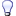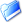Ham Radio
Ham Radio, Electronics, Software and more...

Home » Computers » Hardware » Dell SK-815 Keyboard Mapping Circuit (Dell SK-815 Keyboard Mapping Circuit)
Dell SK-815 Keyboard Mapping Circuit Sat, 25 March 2017 15:06root Messages: 208Registered: December 2004 Senior Member
This will be used to retrofit a laptop keyboard for the Raspberry Pi or the Pine64 inside a laptop.Touching several pins together will give you a key combinations.

These are the results so far:
25 to 10 = delete
25 to 13 = f1
24 to 7 = right arrow and 6 when numlock
24 to 10 = left arrow
24 to 13 = caps lock
24 to 14 = f3
24 to 15 delete
24 to 16 = t
24 to 17 = y
24 to 18 = ]
23 to 13 = \
23 to 8 = up
23 to 7 = delete
22 to 8 = end
22 to 13 = 2
21 to 3 = z
21 to 4 = q
21 to 5 = a
20 to 2 = /
20 to 3 = \
20 to 4 = p
20 to 5 = ;
19 to 3 = .
19 to 4 = o
19 to 5 = l
18 to 3 = ,
18 to 4 = i
18 to 5 = k
17 to 2 = n
17 to 3 = m
17 to 4 = u
17 to 5 = j
17 to 22 = 7
17 to 23 = h
17 to 24 = y
17 to 25 = 6
16 to 3 = v
16 to 4 = r
16 to 5 = f
16 to 22 = 4
16 to 23 = g
16 to 24 = t
16 to 25 = 5
16 to 2 = b
15 to 2 = space
15 to 3 = return
15 to 5 = \
14 to 3 = c
14 to 4 = e
14 to 5 = d
13 to 3 = x
13 to 4 = w
13 to 5 = s
12 to 3 = shift (probably 12 only)
11 to 3 = start menu
10 to 2 = down arrow
9 to 2 = right arrow
9 to 3 = /
9 to 4 = 8
9 to 5 = 2
10 to 4 = home
10 to 5 = end
9 to 5 = arrow down
8 to 2 = left arrow
8 to 5 = enter
8 to 4 = +
4 to 6 = right click
4 to 7 = page up
3 to 7 = *
3 to 10 = numlock
2 to 7 = -
2 to 9
1 to 2 = f10

[Updated on: Sat, 25 March 2017 17:50]

Report message to a moderatorDell SK-815 Keyboard Mapping Circuit By: root on Sat, 25 March 2017 15:06
 Previous Topic: Fixed: A disk read error occurred. Press Ctrl+Alt+Del to restart
Goto Forum:

Current Time: Sat Oct 01 08:09:59 EDT 2022

Total time taken to generate the page: 0.03700 seconds